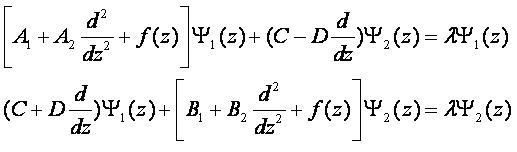# eigenvalue

## Bathe's subspace iteration, how to find the largest eigenvalue/mode?

Does anyone know how to modify Bathe's subspace iteration eigensolver to compute the highest eigenvalue instead of the smallest ?

## Math Kernel Library MKL

Choose a channel featured in the header of iMechanica:

I want to use Intel Math Kernel Library (MKL) to calculate the bifurcation loads and modes in my custom FE program. MKL has many algorithms and I want to use the most efficient one.
Basically, I need to find a few eigenvalues lambda_i and eigenvectors u_i for the problem
KG * u = lambda * KS * u
where KG is the stiffness matrix and KS is the geometric stiffness matrix, also called stress-displacement matrix.
Is KG supposed to be positive definite?

## Bifurcation Analysis in Abaqus

Hello Everyone,

Im relatively new to Abaqus and I have to carry out a bifurcation analysis of a pipe. Once that is done I need to add the imperfection wavelength and amplitude calculated to the pipe and carry out further simulations.

Ive been trying very hard to get a direction as to where to start with it. Can anyone please help me with it? I know that you need to carry out a buckle analysis that will give you a wrinkle pattern on the pipe. This inturn will add up to the bifurcation scheme that you get.

## principal directions for 4th order tensor

Is it possible to find eigenvalues and principal directions for a 4th order tensor? How?

For a zero order tensor? for a first order tensor? for a third order tensor.........

many thanks

## Find eigenvalues of the coupled differential equations by Finite Difference Method.

Hello everybody,

The coupled differential equations need to solve as following:Could you help me to discretize this systems and find the eigenvalue λ  numerically using finite difference method?

lqkhai